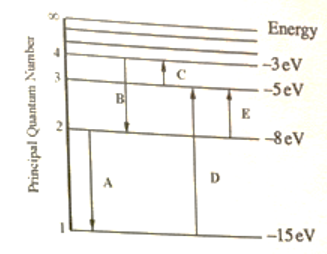# Problem: For this question consider the figure that shows an energy level diagram for a certain atom (not hydrogen). Several transitions are shown and are labeled by letters. Note: The diagram is not drawn to scale. Which transition corresponds to the emission of a 177 nm photon? Which transition corresponds to the absorption of a 124 nm photon?

###### FREE Expert Solution

We’re being asked to determine transition corresponding to the emission of 177 and absorption 124 nm photon.

Recall that the energy of a photon (E) is given by:

where:

h = Planck’s constant (6.626 × 10–34 J • s)

v = frequency (in s–1)

Also, recall that the frequency (v) and wavelength (λ) are related:

where:

c = speed of light (3.0 × 108 m/s)

91% (268 ratings)###### Problem Details

For this question consider the figure that shows an energy level diagram for a certain atom (not hydrogen). Several transitions are shown and are labeled by letters. Note: The diagram is not drawn to scale.Which transition corresponds to the emission of a 177 nm photon?

Which transition corresponds to the absorption of a 124 nm photon?